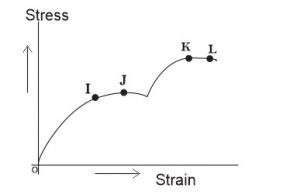## Physics 2019 Past Questions | JAMB

Study the following Physics past questions and answers for JAMBWAEC  NECO and Post-JAMB. Get prepared with official past questions and answers for upcoming examinations.

21. When the downward current flows in a straight vertical conductor, the direction of its magnetic field at a point due north of the wire is

• A. Upward
• B. North
• C. South
• D. West

22. Aluminium is sometimes used as the leaf of an electroscope because it

• A. is a light material
• B. is a good conductor
• C. is a good insulator
• D. can be converted into thin sheets

23.The diagram above represents the stress-strain graph of a loaded wire. Which of these statements is correct?

• A. At J, the wire becomes plastic
• B. J is the yield point
• C. L is the elastic limit
• D. At K, the wire breaks

24.  A supply of 400V is connected across capacitors of 3μf and 6μf in series. Calculate the charge

• A. 8 x 10$$^{-4}$$C
• B. 4 x 10$$^{-2}$$C
• C. 8 x 10$$^{-3}$$C
• D. 4 x 10$$^{-8}$$C

25. A vibrator causes water ripples to travel across the surface of a tank. The wave travels 50cm in 2s and the distance between successive crests is 5cm. Calculate the frequency of the vibrator

• A. 5Hz
• B. 6Hz
• C. 5.2Hz
• D. 25Hz

26. A thermocouple thermometer is connected to a millivoltmeter which can read up to 10mV. When one junction is in ice at 0°C and the other is steam at 100°C, the millivoltmeter reads 4mV. What is the maximum temperature which this arrangement can measure

• A. 100${}^{o}$C
• B. 248${}^{o}$C
• C. 250${}^{o}$C
• D. 350${}^{o}$C

27. An alternating current can induce voltage because it has

• A. ripple value
• B. varying magnetic field
• C. weaker magnetic field than direct current
• D. high peak value

28. The limiting frictional force between two surfaces depends on

I. the normal reaction between the surfaces
II. the area of surface in contact
III. the relative velocity between the surfaces
IV. the nature of the surfaces

• A. I only
• B. II only
• C. III only
• D. I & IV only

29. During the transformation of matter from the solid to the liquid state, the heat supplied does not produce a temperature increase because

• A. the heat energy is quickly conducted away
• B. the heat capacity has become very large as the substance melts
• C. the heat gained is equal to the heat lost by the substance
• D. all the heat is used to break the bonds holding the molecules of the solid together

30. The statement ‘Heat lost by the hot body equals that gained by the cold one’ is assumed when determining specific that heat capacity by the method of mixtures. Which of the following validates the assumption?

I. Lagging the Calorimeter
II. Ensuring that only S.I units are used
III. Weighing the calorimeter, the lid and the stirrer.

• A. I only
• B. I & II only
• C. I & III only
• D. II & III only
Subscribe
Notify ofInline Feedbacks